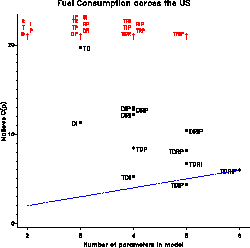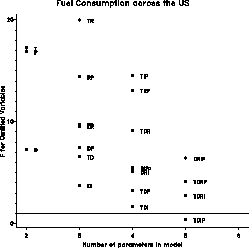cpplot C(p) plots for model selection cpplot

# SAS Macro Programs: cpplot

\$Version: 1.5-1 (27 Feb 2013)
Michael Friendly
York University

## The cpplot macro (get cpplot.sas)

### Plots of Mallow's C(p) and related statistics for model selection

The CPPLOT macro plots of Mallow's C(p) and related statistics for model selection in linear models. In a graph of C(p) vs. p, good models are those for which C(p) <= p. The program optionally plots other equivalent statistics for which the reference line for good models is horizontal.
• (C(p) / p) vs. p. The reference value for good models is at (C(p)) / p = 1. This is referred to as the CD plot in the GPLOT= and PPLOT= parameters
• F(p) vs. p, where F(p) is the partial F statistic for testing significance of the variables omitted from the model. The reference value for good models is at F(p) = 1. This is referred to as the F plot in the GPLOT= and PPLOT= parameters
• Prob ( F > F(p) ) vs. p, plotted on a log scale. This turns the plot scale around, so that good models are highest on the vertical scale. This is referred to as the FPROB plot in the GPLOT= and PPLOT= parameters
• AIC vs. p, where AIC is Akaike's Information Criterion. Good models are those lowest on the vertical scale. This is referred to as the AIC plot in the GPLOT= and PPLOT= parameters
The program produces a high-resolution plot of C(p) by default. Optionally, it can produce printer plots in any of the above forms. These are useful only with SAS Version 6.07 or later, where PROC PLOT can label points with character strings.

### Method

The macro uses PROC REG with / SELECTION=RSQUARE on the MODEL statement, and extracts the values to be plotted using the OUTEST= option.

cpplot uses the label macro to label points in these plots.

## Usage

cpplot is a macro program. Values must be supplied for the YVAR= and XVAR= parameters

The arguments may be listed within parentheses in any order, separated by commas. For example:

```   %cpplot(YVAR=dependentvar, XVAR=predictorvars, ..., )
```

### Parameters

YVAR=
The name of the dependent variable
XVAR=
A list of potential independent variables in the model
DATA=_LAST_
The name of input data set
PLOTCHAR=1 2 3 4 5 6 7 6 8 9 0
Symbols used to identify the independent variables included in any model in the plot. Usually one would specify the first character of the name of each independent variable, in the order listed in XVAR. The PLOTCHAR list is parsed as blank-delimited words, so each symbol may consist of more than one character. However, blanks are removed from the symbol used to identify a particular model.
OPTIONS=
Other options for the MODEL statement, e.g., OPTIONS=AIC to print AIC values.
GPLOT=CP
High-resolution (PROC GPLOT) plots: Specify a list of any one or more of CP CD F PROBF (separated by blanks).
PPLOT=NONE
Printer plots: any one or more of CP CD F PROBF
CPMAX=30
Maximum value of C(p) plotted. Since values of C(p) can be extremely high for unreasonable models, use this parameter to restrict the plot to the more interesting range of models for which C(p) &le CPMAX. Any models with greater values of C(p) are shown off-scale, labelled in red.
FMAX=30
Maximum value of F plotted
NAME=CPPLOT
Name for the graphic catalog entry
GOUT=
Name of the graphics catalog in which the plot is to be stored. Default: WORK.GSEG.

### Example

The example produces C(p) and F plots of models predicting FUEL consumption from all subsets of the predictor variables TAX, DRIVERS, ROAD, INCome, and POPulation.
```%include data(fuel) ;
%include macros(cpplot);       * or, store in autocall library;
%cpplot(data=fuel,
yvar=fuel,
xvar=tax drivers road inc pop,
gplot=CP F, plotchar=T D R I P,
cpmax=20, fmax=20 );
```### See also

boxcox Power transformations by Box-Cox method
label Create Annotate dataset to label observations
inflplot Influence plot for regression models
partial Partial regression residual plots